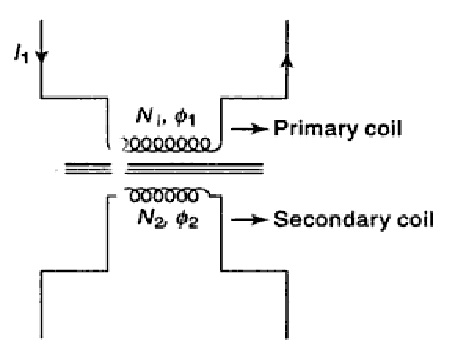## Mutual Inductance

In this post, we will cover Mutual Inductance Definition and Mutual Inductance Formula that will help you to understand Mutual Inductance better.

### Mutual Inductance Definition-

Whenever a change in current occurs in a coil, an induced emf is set up in the neighboring coil. This process is called mutual induction. The coil in which the emf is induced is called the secondary coil.Explanation of Mutual Induction

If a current I(1) flows in the primary coil, the magnetic flux linked with the secondary coil will be-
where M is called mutual inductance between the two coils or circuits.

### Mutual Inductance Formula-

The electromotive force induced in the secondary coil is given by-
Thus the mutual inductance of a pair of circuits is 1 H if a rate of change of current of one ampere per second induces an emf of I V in the other circuit.

Share: